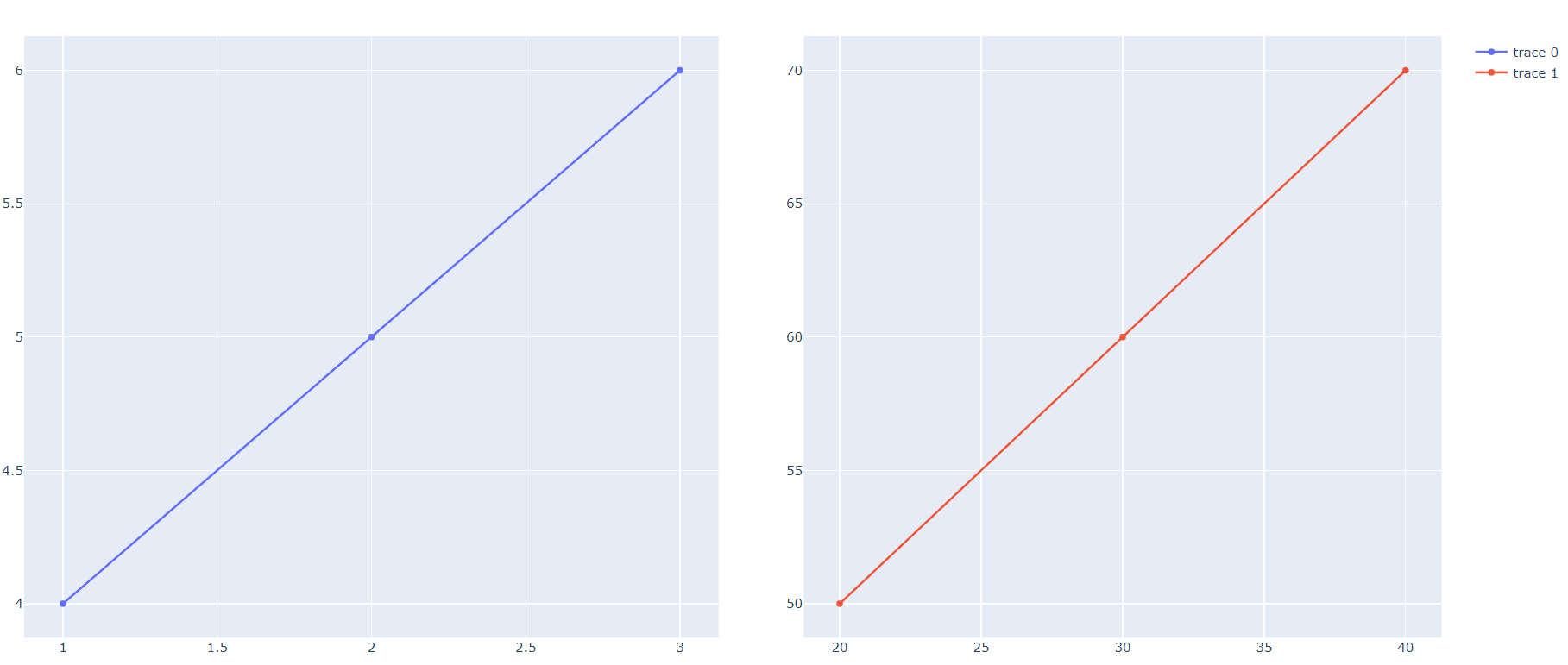# Plotly subplot problem

Member Posts: 9Contributor II

import pandas as pd

#from plotly.subplots import make_subplots

import plotly.graph_objs as go

import plotly

# rm_main is a mandatory function,

# the number of arguments has to be the number of input ports (can be none)

def rm_main():

fig = plotly.tools.make_subplots(rows=1, cols=2)

go.Scatter(x=[1, 2, 3], y=[4, 5, 6]),

row=1, col=1

)

go.Scatter(x=[20, 30, 40], y=[50, 60, 70]),

row=1, col=2

)

fig['layout']['xaxis{}'.format(1)].update({'domain': [0, 0.49]})

plotly.offline.plot(fig)

This is not creating subplot . Howwver jupyter notebook is creating subplot.

I am using RM 9.2

plotly 3.6.1

• Moderator, Member Posts: 1,207Unicorn
Hello @MohammedHasan_G

You should add some statements in the code. Try the below XML code in your Rapidminer. Tou use the below XML code, copy the code from below and paste in your XML window and click on green tick mark, You can see the process. If you cannot find XML window go to View --> Show Panel --> XML. Also, do not forget to change the repository entry to a location where you want to save this plot in "write file" operator.

XML Code: (Click on Show)

<?xml version="1.0" encoding="UTF-8"?><process version="9.3.001">
<context>
<input/>
<output/>
<macros/>
</context>
<operator activated="true" class="process" compatibility="9.3.001" expanded="true" name="Process">
<parameter key="logverbosity" value="init"/>
<parameter key="random_seed" value="2001"/>
<parameter key="send_mail" value="never"/>
<parameter key="process_duration_for_mail" value="30"/>
<parameter key="encoding" value="SYSTEM"/>
<process expanded="true">
<operator activated="true" class="python_scripting:execute_python" compatibility="9.3.000" expanded="true" height="82" name="Execute Python" width="90" x="179" y="34">
<parameter key="script" value="import pandas as pd&#10;import matplotlib.pyplot as plt&#10;#from plotly.subplots import make_subplots&#10;&#10;import plotly.graph_objs as go&#10;&#10;import plotly&#10;&#10;# rm_main is a mandatory function,&#10;&#10;# the number of arguments has to be the number of input ports (can be none)&#10;&#10;def rm_main():&#10;&#9;fig = plotly.tools.make_subplots(rows=1, cols=2)&#10;&#9;fig.add_trace(go.Scatter(x=[1, 2, 3], y=[4, 5, 6]),row=1, col=1)&#10;&#9;fig.add_trace(go.Scatter(x=[20, 30, 40], y=[50, 60, 70]),row=1, col=2)&#10;&#9;fig['layout']['xaxis{}'.format(1)].update({'domain': [0, 0.49]})&#10;&#9;plotly.offline.plot(fig)&#10;&#9;path = &quot;PlotTest.png&quot;&#10;&#9;plt.savefig(path)&#10;&#9;myfile = open(path,'r')&#10;&#9;return(myfile)"/>
<parameter key="notebook_cell_tag_filter" value=""/>
<parameter key="use_default_python" value="true"/>
<parameter key="package_manager" value="conda (anaconda)"/>
</operator>
<operator activated="true" class="write_file" compatibility="9.3.001" expanded="true" height="68" name="Write File" origin="GENERATED_TUTORIAL" width="90" x="581" y="136">
<parameter key="resource_type" value="repository blob entry"/>
<parameter key="repository_entry" value="//Local Repository/data/Plot"/>
<parameter key="mime_type" value="image/png"/>
</operator>
<connect from_op="Execute Python" from_port="output 1" to_op="Write File" to_port="file"/>
<connect from_op="Write File" from_port="file" to_port="result 1"/>
<portSpacing port="source_input 1" spacing="0"/>
<portSpacing port="sink_result 1" spacing="0"/>
<portSpacing port="sink_result 2" spacing="0"/>
</process>
</operator>
</process>

Result:Hope this helps.
Regards,
Varun
https://www.varunmandalapu.com/

# Be Safe. Follow precautions and Maintain Social Distancing

• Member Posts: 9Contributor II
Thanks for the answer. I got the plot. However, I am confused about the code. Actually I need the html file with interactive plot. I do not need the png file. Here I can see you are creating png file and saving in the directory.

I tried this code. Because I don't need the png file and it worked.

def rm_main():
fig = plotly.tools.make_subplots(rows=1, cols=2)
fig.add_trace(go.Scatter(x=[1, 2, 3], y=[4, 5, 6]),row=1, col=1)
fig.add_trace(go.Scatter(x=[20, 30, 40], y=[50, 60, 70]),row=1, col=2)
fig['layout']['xaxis{}'.format(1)].update({'domain': [0, 0.49]})
plotly.offline.plot(fig, filename = 'hhh.html')
path = "PlotTest.png"
plt.savefig(path)
#myfile = open(path,'r')
#return(myfile)

However I tried to comment these two lines
path = "PlotTest.png"
plt.savefig(path)

Why without these two lines, the html file is not found. Can you please clarify me, what is the relation between creating html file and png file in case of subplot. I mean why I need to add these two extra lines in case of subplot

I also mentioned, This problem happened during only subplot. Normal html plot I can create without any problem (means without these two lines).